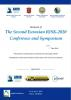# Mathematical Foundations of Risk Analysis

## Authors

Nozer Singpurwalla
The George Washington University, Emeritus Professor of Statistics

### Synopsis

The mathematical theory of risk analysis rests on two pillars and one principle. The two pillars are probability and utility, and the principle is the maximization of expected utility (MEU). The latter is a consequence of the calculus of probability, particularly, the law of total probability. The law of total probability is germane here because of the argument that utilities are like probabilities and thus obey the laws of probability.Published
April 12, 2020